Want to share your content on R-bloggers? click here if you have a blog, or here if you don't.

# Introduction

We are deprecating `fitPlot()` from the next version of FSA (v0.9.0). It will likely be removed at the end of the year 2001. We are taking this action to make `FSA` more focused on fisheries applications and to eliminate “black box” functions. `fitPlot()` was originally designed for students to quickly visualize the results of one- and two-way ANOVAs and simple, indicator variable, and logistic regressions.1

We now feel that students are better served by learning how to create these visualizations using methods provided by `ggplot2`, which require more code, but are more modern, flexible, and transparent.

The basic plots produced by `fitPlot()` are recreated here using `ggplot2` to provide a resource to help users that relied on `fitPlot()` transition to `ggplot2`.

The examples below require the following additional packages.

Most examples below use the `Mirex` data set from `FSA`, which contains the concentration of mirex in the tissue and the body weight of two species of salmon (`chinook` and `coho`) captured in six years. The `year` variable is converted to a factor below for modeling purposes and a new variable is created that indicates if the mirex concentration was greater that 0.2 or not. This new variable is used to demonstrate a logistic regression.

# One-Way ANOVA

The code below fits a one-way ANOVA model to examine if mean weight differs by species.

There are at least two simple ways to visualize results from a one-way ANOVA. First, summarized means of raw data with 95% confidence intervals derived from the standard deviation, sample size, and degrees-of-freedom specific to each group are shown.

Second, marginal means may be predicted or estimated from the fitted model (discussed in detail in this vignette from the `emmeans` package). However, the main difference from above is that the confidence intervals for the marginal means use an “overall” standard deviation and degrees-of-freedom estimated from across all groups. The predicted or estimated marginal means may be computed with `predict()`

or, equivalently, with `emmeans()` from the `emmeans` package …

`fitPlot()` from `FSA` (before v0.9.0) uses the first method to display means with 95% confidence intervals for both species.### Using Manually Summarized Means

The summarized means saved in `sumdata` above can be plotted as shown below to recreate the `fitPlot()` result. Note `width=0.1` in `geom_errorbar()` is used to reduce the width of the “caps” at the confidence values and `group=1` is needed in `geom_line()` as there is only one point for each factor level. Changes (themes, colors, labels, etc) to this basic plot can be made as usual for `ggplot()`s (and is illustrated further below).### Using Built-In Functions for Summarized Means

This plot can also be created using `stat_summary()` from `ggplot` coupled with `mean_cl_normal()` and `mean()`. Take note of how each `geom=` in each `stat_summary()` mirrors what was used above. Also note the use of `width=0.1` and `group=1` here as done above.### Using Marginal Means from `emmeans`

The estimated marginal means may be plotted similarly to the manually summarized means but by using the `aov1mcs\$emmeans` object created above.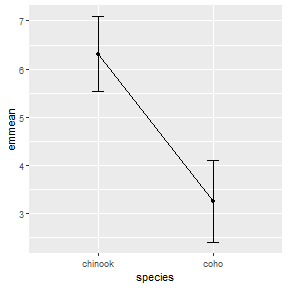# Two-Way ANOVA

The code below fits a two-way ANOVA model to examine if mean weight differs by species, by year, or by the interaction between species and year.

The `fitPlot()` from `FSA` (before v0.9.0) shows the mean with 95% confidence interval for all combinations of species and year.### Using Built-In Functions for Summarized Means

Again, the `stat_summary()` function can be used to efficiently calculate and then plot the 95% confidence intervals and means similar to what was shown above for a one-way ANOVA. However, there are three major differences.

First, in the main `ggplot()` call the color of the points and lines is mapped to one of the two factor variables (`species` in this case) whereas the other factor variable is mapped to `x=`.2 Second, the `group=` aesthetic for the line geom must be set to the factor that describes how the lines should be connected (e.g., `species` in this case). Third, the intervals and (possibly) the points at each level on the x-axis will overlap if they are not “dodged” a small amount.3 The “dodge” amount should be set outside the `geom()`s so that each geom uses the same amount of “dodging.” This will assure that the intervals, points, and connecting lines for each level defined by the colors align. Below this “dodge” amount is set with `position_dodge()` and saved to an object called `pd` which is then set equal to `position=` in each `geom()`.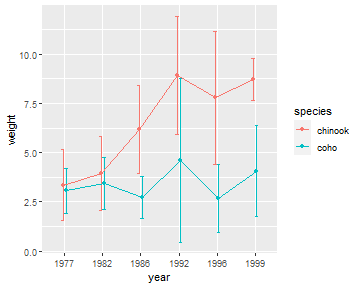### Using Marginal Means from `emmeans`

The plot with marginal means is constructed similarly as shown below. Note, however, `year:species` in `emmeans()` is used so that the marginal means and confidence intervals are estimated for each combination of `year` and `species`.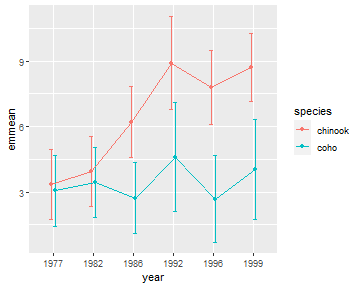# Simple Linear Regression

The code below fits a simple linear regression for examining the relationship between mirex concentration and salmon weight.

`fitPlot()` from `FSA` (before v0.9.0) shows the best-fit line with a 95% confidence band.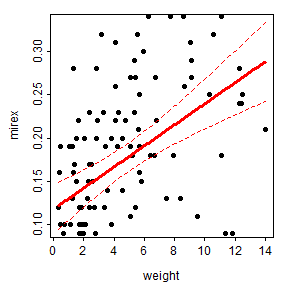### Using Manually Predicted Values

One method for recreating this plot is to create a new data frame that first has the two variables of observed data and then adds on predicted values of the response at each observed value of the explanatory variable with 95% confidence intervals. The two observed variables are selected from the original data frame with `dplyr::select()`. If this new data frame is given to `newdata=` in `predict()` with `interval="confidence"` then the predicted values (and 95% confidence intervals) will be constructed at each value of the explanatory variable. The two data frames are then column-bound together with `cbind()` to make one data frame for plotting (further below).

The confidence band is first plotted as a “ribbon” with the best-fit line then added followed by the observed points. In this plot, `weight` is globally mapped to `x=` in `ggplot()` so that it will be used for each geom. The lower and upper confidence values to `ymin=` and `ymax=` in `geom_ribbon()`, whereas the predicted or “fit”ted values are mapped to `y=` `geom_line()` to make the line and the observed mirex concentrations are mapped to `y=` in `geom_point()` to plot the observed points. Further note the use of `alpha=` to make the confidence band semi-transparent and `size=` to make the fitted line slightly larger than the default. Again all aspects of this plot can be changed in the usual ggplot way.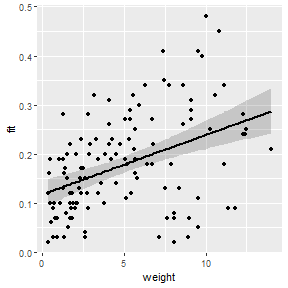### Using A Built-In Function

The best-fit line can also be added to a scatterplot with `geom_smooth()`.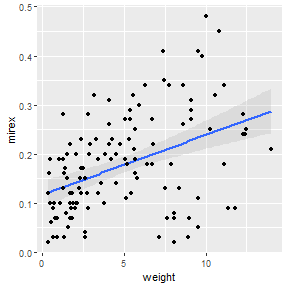## Indicator Variable Regression

The code below fits an indicator variable regression to examine if the relationship between mirex concentration and salmon weight differs betwen species.

The `fitPlot()` from `FSA` (before v0.9.0) shows the best-fit line for both species.### Using Manually Predicted Values

The process of constructing a similar plot in `ggplot()` follows the same general procedure as that for a simple linear regression. First, make a data frame that has the observed variables used in the model and predicted values and confidence limits for each observation.

Then plot the data as before but making sure to map `color=` and `fill=` (just for the ribbon) to the species factor variable.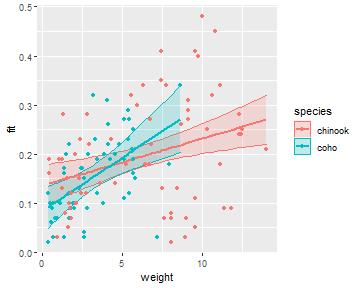### Using a Built-In Function

This plot can also be constructed with `geom_smooth()`, again making sure to map the `color=` and `fill=` to the species factor variable.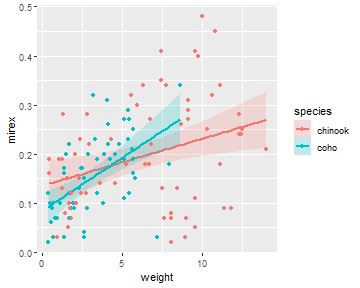## Logistic Regression

The code below fits a logistic regression to examine the relationship between the probability that mirex concentration is greater than 0.2 and salmon weight.

`fitPlot()` from `FSA` (before v0.9.0) shows the fitted logistic regression curve.### Using Manually Predicted Values

The first method for showing the logistic regression curve follows the general methodology for simple linear regression shown above. Note, however, that `predict()` does not produce confidence interval values for a logistic regression. Thus, the plot created in this way cannot have a confidence band.### Using a Built-In Function

The best-fit logistic regression curve with a confidence band can, however, be added to a scatterplot with `geom_smooth()`. In this case, `method=` must be changed to `glm` and `method.args=` must be used as shown below so that `glm` will construct a logistic (rather than linear) regression.Note that this method easily generalizes to an indicator variable logistic regression (note that `color=` and `fill=` are mapped to the species factor variable).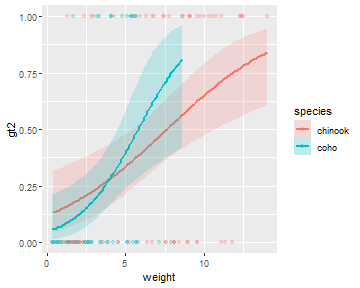## Polynomial Regression

The code below fits a quadratic (second degree polynomial) regression for the relationship between mirex concentration and salmon weight.

`fitPlot()` from `FSA` (before v0.9.0) shows the best-fit regression curve.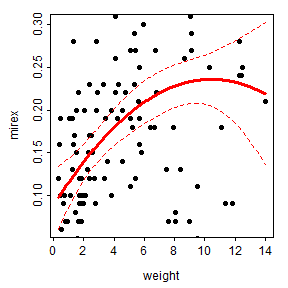### Using Manually Predicted Values

This regression can be viewed similarly to the way the simple linear regressions was viewed.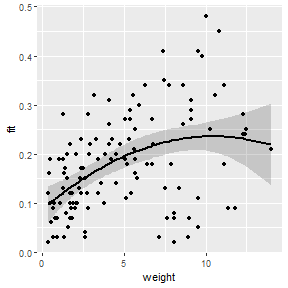### Using a Built-In Function

This type of regression can also be viewed using `geom_smooth()` but the formula for the polynomial must be given to `formula=`. However, note that in this formula you put `y` and `x` rather than the names of the variables that are mapped to `y` and `x`.## Nonlinear Regression

The following code fits a von Bertalanffy growth function (VBGF) to the total length and age data for spot found in the `SpotVA1` data frame built into `FSA`. Fitting the VBGF is described in more detail here.

`fitPlot()` from `FSA` (before v0.9.0) shows this model fit to the observed data.

### Using Manually Predicted Values

A similar plot can be constructed from manually predicted values very similarly to what was described for previous models. However, as with the logistic regression, `predict()` will not return confidence interval values, so it is not immediately possible to create a confidence band with this method.### Using a Built-In Function

You can get a very similar plot using `geom_smooth()` with `formula=` set equal to the same formula as in `nls()` (except using `y` and `x` rather than the variables mapped to those aesthetics) and putting the `start=` argument in the `method.args=` list. Note that the algorithm used by `geom_smooth()` to computed the confidence bands will err for this non-linear model; thus, set `se=FALSE` to at least see the best-fit curve.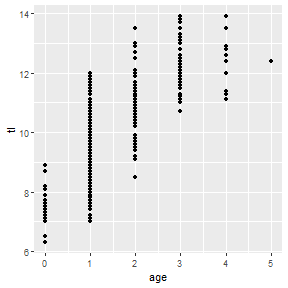Make a similar plot but with a confidence band derived via bootstrapping is described in this previous post.

## Conclusion

The `fitPlot()` function in `FSA` will be deprecated in v0.9.0 and will likely not exist after that. This post describes a more transparent (i.e., not a “black box”) and flexible set of methods for constructing similar plots using `ggplot2` for those who will need to transition away from using `fitPlot()`.

As mentioned in the examples above, each plot can be modified further using typical methods for `ggplot2`. These changes were not illustrated above to minimize the amount of code shown in this post. However, as an example, the code below shows a possible modification of the IVR plot shown above.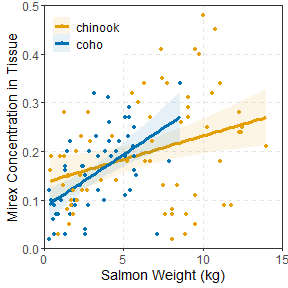## Footnotes

1. Over time functionality for non-linear regressions was added.

2. These two variables can, of course, be exchanged. However, I generally prefer to have the variable with more levels on the x-axis.

3. Note this same problem occurs for the `fitPlot()`, though there is no simple solution for it.